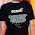## Wednesday, December 23, 2009

### SOLUTION TO MECHANICAL BOARD EXAM PROBLEMS: Power Set-1

Here are the solutions to board problems given at Power Subject Set-1. If you do not understand anything, feel free to write a comment voicing out your concern. I'm still looking for better ways to present my solution. I still don't know how to present equations and mathematical symbols with blogger. Please bear with me.

1. An automobile tire is inflated to 32psig pressure at 50 degree F. After being driven, the temperature rises to 75 degree F. Determine the final gage pressure assuming the volume remains constant. (Electrical Engineering Board Exam Problem)

Solution:
Since volume is constant, use Charles’ Law on constant volume processes.
P1/T1=P2/T2
Note: P and T should be absolute.

(32+14.7)/ (50+460) =P2/ (75+460)

P2=48.99 psia

Converting back to gage pressure,

P2g=48.99-14.7

P2g=34.29 psig

2. Four hundred cubic centimeters of gas at a pressure of 740 mmHg absolute and temperature of 18 degree C undergoes a process until the pressure and temperature becomes 760 mmHg absolute and 0 degrees C respectively. Calculate the final volume of the gas. (Electrical Engineering Board Exam Problem)

Since all the parameters (temperature, pressure and volume) changes, use combined gas law

PV/T=constant

P1V1/T1=P2V2/T2

(740)(400)/(18+273)=(760)V2/(0+273)

V2=365.3 cc

3. A single acting air compressor with a clearance of 6% took air at atmospheric pressure and temperature of 85 degrees F, discharges it at a pressure of 85 psia. Assuming that the compression is isentropic, find the piston displacement per cycle and the air power (hp) of the compressor if the RPM is 750. (Mechanical Engineering Board Exam 1978)
(I haven't got this correct yet, LOL)

4. Steam enters turbine stage with an enthalpy of 3628 kJ/kg at 70m/s and leaves the same stage with an enthalpy of 2846 kJ/kg and velocity of 124 m/s. calculate the work done by the steam. (Mechanical Engineering Board Exam 1986)

Solution:

Just like the 90% of all thermo problems, this one can be solved using the first law of thermodynamics.

Ein=Eout

H1+KE1=H2+KE2+W

W=(H1-H2)+(KE1-KE2)

W=(3628-2846)+(70^2/1000-124^2/1000)
W=776.5 76J/kg

5. In an air standard Otto cycle, the clearance volume is 18% of the displacement volume. Find the thermal efficiency? (Mechanical Engineering Board Exam 1993)

Solution:

e = 1-1/rk^(k-1)

rk = (1+c)/c = (1+.18)/0.18 = 6.556

e = 1-1/(6.6556)^(1.4-1)=.53

e=53%

6.A reversed Carnot cycle is used for cooling. The input work is 12kW, while the COP is 3.8. Calculate the refrigerating effect in TOR. (Mechanical Engineering Board Exam)

Solution:

COP=Refrigerating Effect/Compressor Work

Refrigerating Effect = COP (Comp Work)

RE=3.8(12kW)(1Tor/3.516kW)=12.97TOR

7. The compression ratio of a standard gasoline engine is 8. The heat added is 1850kJ/kg. If the initial pressure and temperature are 100Kpa and 25 degrees C respectively, determine the temperature at the end of isentropic expansion. (Mechanical Engineering Board Exam)

Solution:

Rk=8

Qa=1850kJ/kg

P1=100kPa

T1=25+273=298K

From the given compression ratio, rk=8

rk=V1/V2=8

T2/T1=(V1/V2)^(k-1); k of air is 1.4

T2=((8)^(1.4-1))(298)

T2=684.62K

Process 2 -3 is constant volume

Qa=cv(T3-T2) ; cv for air is 0.7186 kJ/kg-K

1850=(.7186)(T3-684.62)

T3=3259.07K

Process 3-4 is isentropic expansion,

V4/V3=rk

(V4/v3)^(k-1)=(T3/T4)

T4 = 3259.07/((8)^(1-1.4))

T4 = 1418.6K

t = 1145.6 deg C

8. What is the logarithmic mean temperature difference (LMTD) of a double pipe counterflow heat exchanger if the fluid enters at 90 degrees C and exits at 50 degrees C. Inside the inner pipe, another fluid enters at 20 degrees C and exits at 60 degrees C. (Mechanical Engineering Board Exam)

Solution:
Since you will get zero when you use the conventional LMTD version, use the arithmetic mean temperature difference (AMTD) instead.

AMTD=((90-60)+(50-20))/2=30

9. The volume of concrete needed for the foundation of an engine is 12 cubic meters. The concrete mixture is 1:3:5 by volume. Calculate the number of 40 kg bags of cement needed considering the density of cement to be 1500kg/cu.m. (Mechanical Engineering Board Exam)

Solution:

This is actually just a mixture problem in algebra:

If the mixture is 1:3:5 by volume, then the volume of cement is

(1/(1+3+5))(12 cu.m)=1.33 cu.m

We will use the cement density given to calculate the mass equivalent of this volume.

m = density(Volume)

m = 1500 (1.33) =2000 kgs

To calculate the number of 40-kg cement sacks,

Sacks=(2000kg)(1sack/40kg)

Sacks=50 sacks

10. A 305mm x 457mm four stroke single acting diesel engine is rated at 150 kW at 260 rpm. Fuel consumption at rated load of 0.26 kg/kw-hr with a heating value of 43912 KJ/kg. Calculate the brake thermal efficiency. (Mechanical Engineering Board Exam 1990)

Eb=Brake Power/Heat Input

Brake Power= 150kW

Heat Input=(Fuel mass)(Heating Value)

Fuel mass=(150kw)(0.26kg/kw-hr)

Fuel mass=39kg/hr

Heat Input=(39)(43912) =1712568kJ/h =475.71 kW

Eb=(150)/(475)=31.53%

Hope this helps.

1.tnk UU>> p000!! godbless

2.thank you

3.panu po kami makakakuha ng mga problems galing sa previous ME board exams?

4.Viair is the only compressor that I will use on any of my air ride installs. I have had the one on my truck last 1 years and it still works. And I think it is only best air compressor

5.Most people don't want to put up with their A/C units being shut off during the hottest part of the day, or their pool motor not running in the afternoon or early evening when they want to use it. Fortunately, when relatives visit me, they understand my OCD about keeping appliances shut off, and just giggle aout me when they get home, so I don't have to worry about them bhttp://homemachine.net

6.I assume this is an informative publicize and it is each useful and knowledgeable. accurately, i'd inside the melody of to thanks for the efforts you have got made in writing this newsletter. MP Patwari Recruitment 2019

7.Great job for publishing such a beneficial web site. Your web log isn't only useful but it is additionally really creative too.

BA 1st Year Result